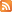Entries (RSS) -Comments (RSS)

### Chapter 3 Foldable

On the front of your foldable list all exponent laws in equally spaced boxes.
Continue these boxes on the inside of your foldable.
On the back of your foldable leave space for the chapter important words.
Leave space for a book definition, a definition in your own words and an example for each word.

Laws:
Product Law
Quotient Law
Power of a Power Law
Power of a Product Law
Power of a Quotient Law
Exponent Law of 1
Negative Exponent Law
Base of 1 Law

Important Words:
power
base
exponent
exponential form
coefficient

Also do Mangahigh and try to learn from these tutorials what some of the laws are.

Have a great break and a Merry Christmas! and a Happy New Year!!

### Chapter 1 Reflection

THINKING ABOUT THINKING
Reflect on Chapter 1 learning outcomes.
Look at page 6, 11; 16, 20; 26 and 31 Focus On and Key Ideas
Tell what learning you did for each section using explanations, diagrams, procedures, meaning of concepts and any relationships you have found.
Explain what things were new to you and how you have grown as a math learner.
What is still bothering you about the unit? In other words, what things did you have difficulty with or are still "fuzzy" about? How do you plan on getting the answers you need?
Explain a concept you are understand and are proud of in this unit.
Explain a concept you had to problem solve and reason about.
Explain how this unit connected to real life for you.
Think about you as a learner… what work did you need to spend more time on? What work is still incomplete? Have you lived up to the goal you set for yourself in your last journal entry to me?
If you have set a goal, do you need to change it? If so do so now. Or recommit yourself to your goal.
If you haven’t set a goal, do so now.

### Elegant Algebraic Equations for 3D prisms

Shape                                      Formula
Cube                                        A = 6s2                            Where s is a side length.

Rectangular Prism                  A = 2B + Ph
Where B is the area of the base, P is the perimeter of the base and
h is the height.

A = 2(lw + lh + wh)
Where l is the length, w is the width,
and h is the height.

Pyramid                                  A = 4[(bh)/2] + lw
Where b is the base length, h is the height of the triangle (the slant
height), l is the length of the base, and w is the width of the base.

A = B + ½(Ph)
Where B is the area of the base, P is the perimeter of the base,
and h is the height of the triangle (the slant height).

Isosceles triangular prism             A = 2[(b1h1)/2]  + 2[(b2h2)/2] + B
Where b1 and h1 is the base and height related to the triangle,
b2 and h2 is the base and height related to the rectangles,

and B is the area of the base.

Cylinder                                          A = 2πr2 + (2πr)h
A = 2πr(r + h)

Where r is the radius of the circle.

Where B is found by finding the area of the base (length x width),
and P is the sum of all sides of the base.

### Homework for Section 1.3

This section is the more difficult section of this unit. It is very important that you spend time time doing many questions in this section. It would help to do all of the questions in the book but you should be able to grasp the concepts by doing the following

Read the pages that explain the work on pages 26-31. Make sure you understand the KEY CONCEPTS
Do all the Show You Knows on these pages.
Do question 2 and 3 in the Check Your Understanding
Do questions 5, 6, 7 and 9 in Practise
Do questions 10, 13, 14, 15, 16, 17, 18 and 19 in Apply
Do questions 22 and 23 in Extend and have an understanding about questions 21 and 20.

The next test will be more difficult than your test. We will probably include some form of translation, reflection or rotation on the test as well.

If you get stuck do not hesitate to ask for help.....

Do not forget your Journals are due tomorrow.